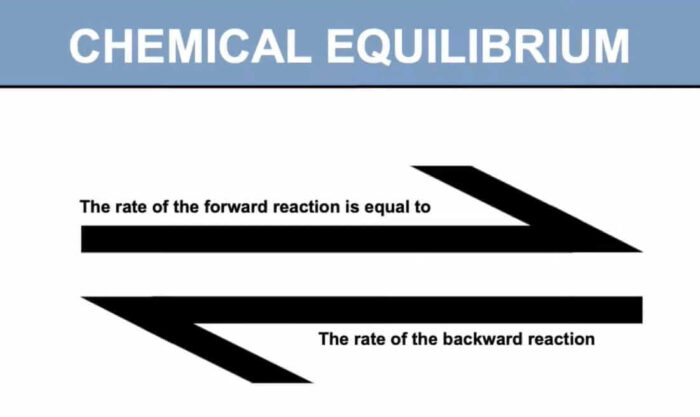In a chemical reaction, chemical equilibrium is the state in which both the reactants and products are present in concentrations which have no further tendency to change with time, so that there is no observable change in the properties of the system. This state results when the forward reaction proceeds at the same rate as the reverse reaction.

## Course Contents

• Chemical equilibria: reversible reactions; dynamic equilibrium
• (i) factors affecting chemical equilibria
• (ii) equilibrium constants
• (iii) the Haber process

## Learning Outcomes

Candidates should be able to:

• (a) explain, in terms of rates of the forward and reverse reactions, what is meant by a reversible reaction and dynamic equilibrium
• (b) state Le Chatelier’s Principle and apply it to deduce qualitatively (from appropriate information) the effects of changes in concentration, pressure or temperature, on a system at equilibrium
• (c) deduce whether changes in concentration, pressure or temperature or the presence of a catalyst affect the value of the equilibrium constant for a reaction
• (d) deduce expressions for equilibrium constants in terms of concentrations, Kc , and partial pressures, Kp [treatment of the relationship between Kp and Kc is not required]
• (e) calculate the values of equilibrium constants in terms of concentrations or partial pressures from appropriate data
• (f) calculate the quantities present at equilibrium, given appropriate data (such calculations will not require the solving of quadratic equations)
• (g) show understanding that the position of equilibrium is dependent on the standard Gibbs free energy change of reaction, ∆G⦵ [Quantitative treatment is not required]
• (h) describe and explain the conditions used in the Haber process, as an example of the importance of an understanding of chemical equilibrium in the chemical industry